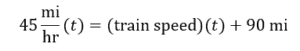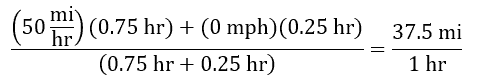# PCAT Quantitative Reasoning – Traveling Distance Word Problem

A vehicle and a train are both traveling directly east at 45 mph and 50 mph, respectively. The train takes a 15-minute stop once an hour to allow new passengers to board. If the vehicle is currently 90 miles behind the train, approximately how long will it take the vehicle to catch up to the train?

1. 2 hours
2. 6 hours
3. 12 hours
4. 18 hours
##### Explanation

C is correct. The very first step to solving a word problem like this should be to quickly sketch it out:Now we can set up the appropriate equation. We want the number of miles that the car travels to equal the number of miles the train travels at the point that the car passes the train. We need the variable t for time, which is what we are solving for. The distance the car travels is its speed (45 mph) times the number of hours it travels (t). We’ll set this equal to the distance that the train travels (the speed of the train times t) plus another 90 miles to account for the extra distance the car must cover. Remember, all the units should yield miles:Why haven’t we plugged in the train’s speed? Well, the train does indeed travel 50 mph when it’s moving. But it’s stationary 25% of the time if it stops for 15 minutes once an hour. So let’s calculate its average speed over the course of an hour if the train travels 50 mph for 45 minutes (0.75 hours) and 0 mph for 15 minutes (0.25 hours):Let’s plug in this average speed back into our original equation and solve:#### Submit a Comment

This site uses Akismet to reduce spam. Learn how your comment data is processed.